# How to Create a Calculated Field in Access

Calculated fields can calculate the values from other fields within a table, as well as from built-in Access functions.

Calculated fields are a special type of field that calculate the values from other fields (or built-in Access functions), then display the result of that calculation. A common example might be to multiply a price field by a quantity field.

In the following example, we will divide a country's population by it's land area. This will give us a population density (i.e. how many people per square kilometer live in that country).

1.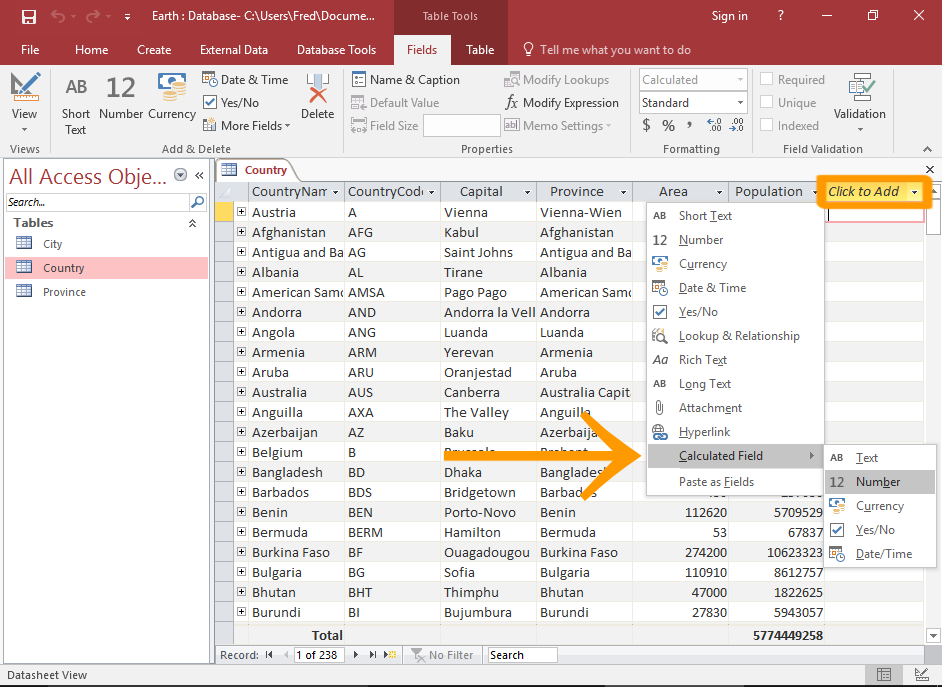Select the Calculated Field Option Open the table in Datasheet View and scroll to the right-most field. Click the Click to Add field heading and select Calculated Field from the drop-down menu, then select the data type that you want for the result. The Expression Builder will launch. You can also initiate this by selecting More Fields > Calculated Field from the Ribbon (on the Fields tab).
2.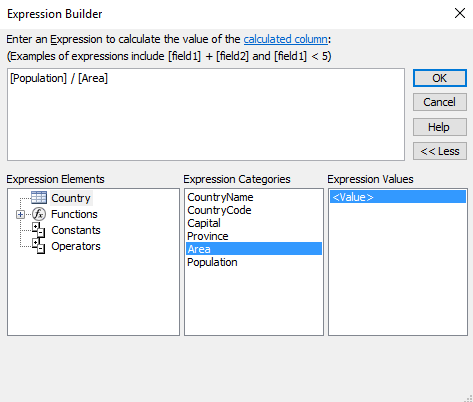Enter an Expression Use the Expression Builder to build the calculation that you want. Or just type the expression if you already know how. In this example, we're dividing the Population field by the Area field. So we type [Population] / [Area]. We're doing this so that we can calculate the number of people per square kilometer. Click OK once you've entered the expression.
3.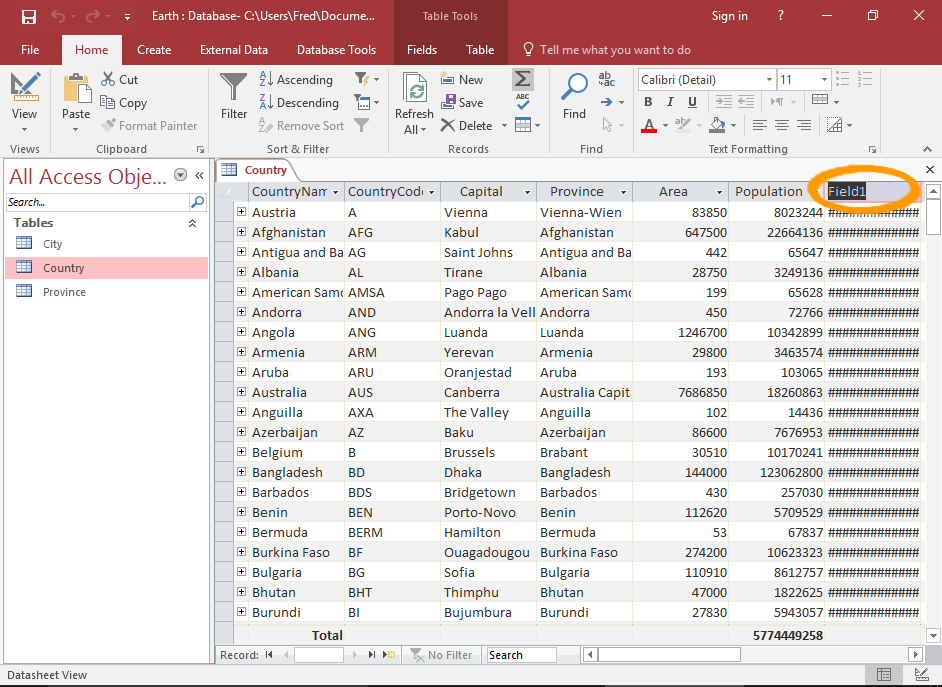Enter a Field Name Access will highlight the field header so that you can enter a name. Go ahead and enter a name for the field.
4.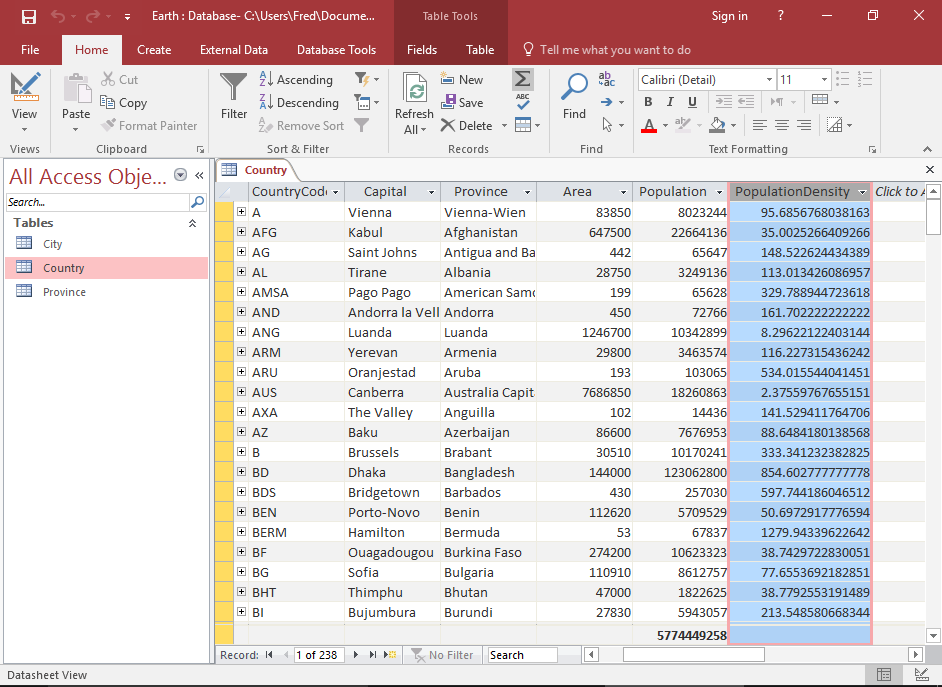Your Calculated Field The calculated field is now complete. You may need to expand the width of the field in order to show the values.

## Modifications

•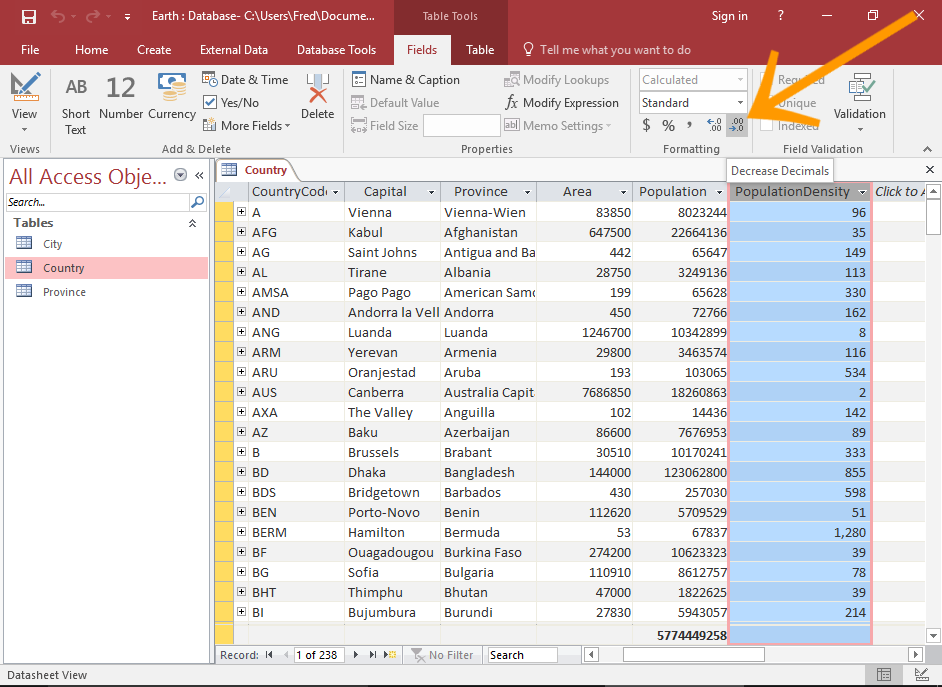Rounding the Numbers If your calculated field provides too many decimal places (or not enough), you can easily adjust this. Simply select the field, then, ensuring that the Fields tab is selected in the Ribbon, click one of the little rounding icons (in the Formatting section of the Ribbon). To decrease the number of decimal places, click the icon on the right. To increase, click the left one.
•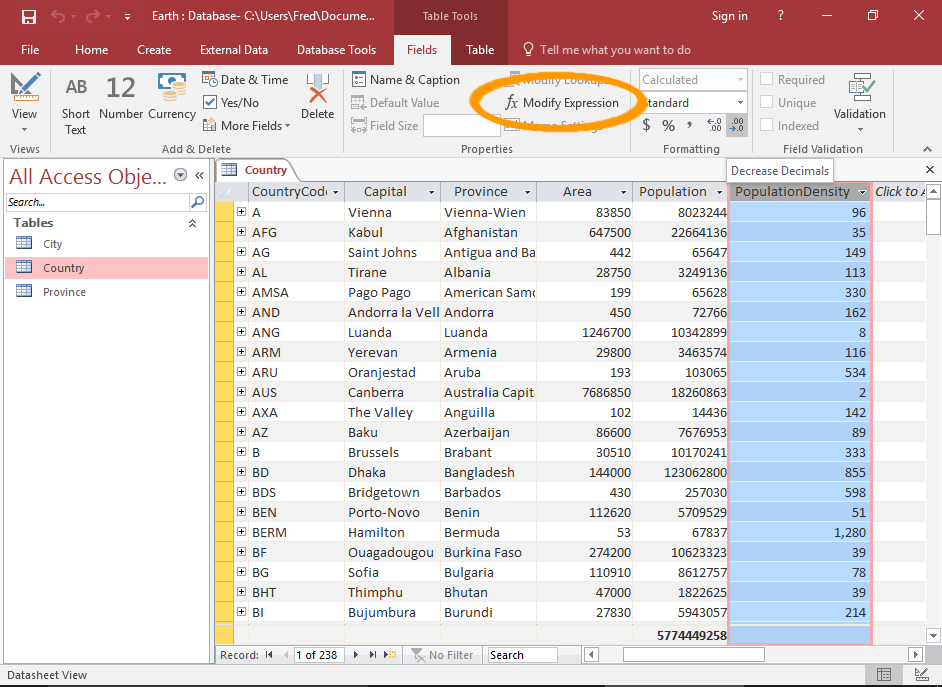Modify the Expression You can launch the Expression Builder if you need to modify the expression. To do this, select the calculated field that you want to modify, then click Modify Expression in the Ribbon (on the Fields tab).